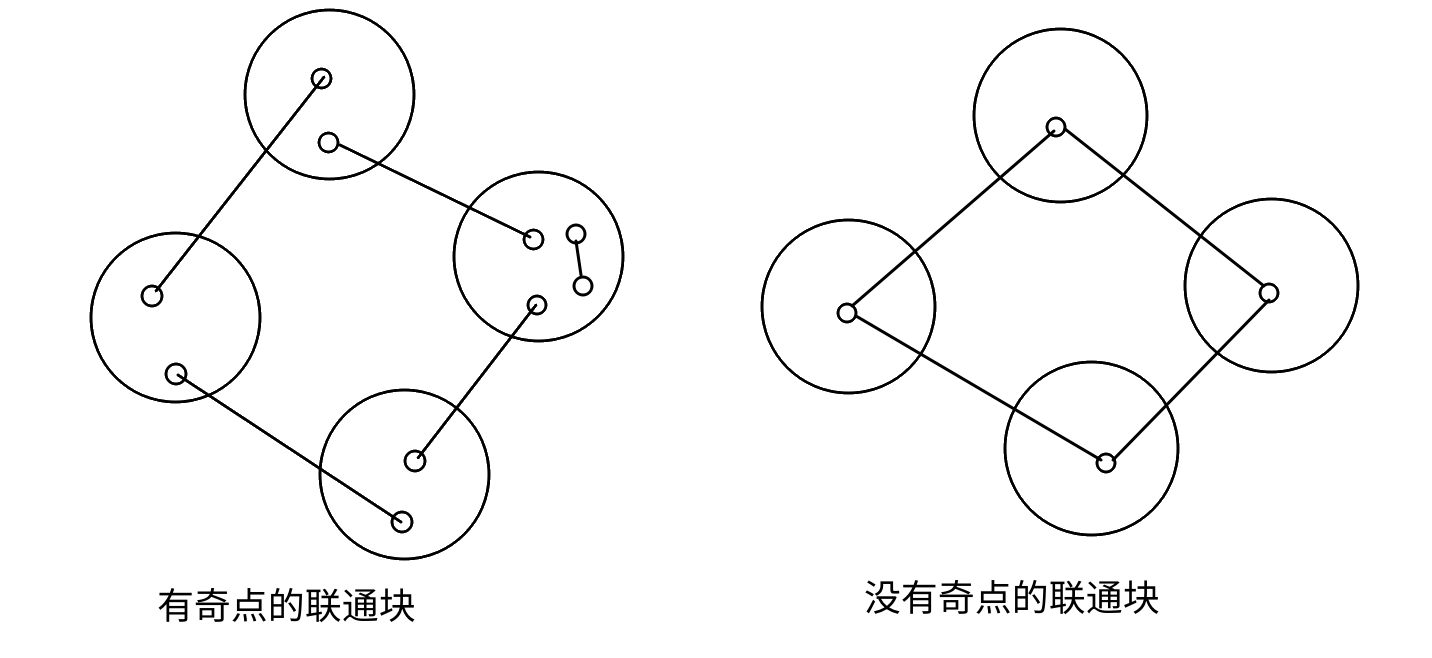2019.6.29

## 欧拉回路

UOJ117

#include<algorithm>/*{{{*/
#include<cctype>
#include<cassert>
#include<cmath>
#include<cstdio>
#include<cstring>
#include<cstdlib>
#include<ctime>
#include<iostream>
#include<map>
#include<queue>
#include<set>
#include<vector>
using namespace std;
typedef long long lld;
typedef long double lf;
typedef unsigned long long uld;
typedef pair<int,int> pii;
#define fi first
#define se second
#define pb push_back
#define mk make_pair
#define FOR(i,a,b) for(int i=(a);i<=(b);++i)
#define ROF(i,a,b) for(int i=(a);i>=(b);--i)/*}}}*/
const int M=1e6+5,N=1e6+5;
int t,n,m;
int a[M],la;

struct qxx{int nex,t,v;};
qxx e[M];
int h[N],le;

bool vis[N];
int d1[N],d2[N];
void dfs(int u){
for(int i=h[u];i;i=h[u]){
while(i&&vis[e[i].v])i=e[i].nex;
h[u]=i;
const int v=e[i].t,w=e[i].v;
if(i)vis[w]=1,dfs(v),a[++la]=i%2?w:(t==2?w:-w);
}
}

int main(){
scanf("%d%d%d",&t,&n,&m);
int u1,v1;
FOR(i,1,m){
scanf("%d%d",&u1,&v1);
d1[u1]++,d2[v1]++;
}
FOR(i,1,n)if((d1[i]&1)^(d2[i]&1)||(t==2&&d1[i]!=d2[i]))return puts("NO"),0;
dfs(u1);
if(la<m)return puts("NO"),0;
puts("YES");
ROF(i,la,1)printf("%d ",a[i]);
return 0;
}

$(x,y)$当作$x,y$之间连一条无向边，则染红色蓝色相当与给无向边定向，转化为上一题的模型。## abs 与 fabs

abs 是憨批。如果不 using namespace std 直接调用 abs 可能调用的是 int abs(int)。使用 fabs 是最保险的。

## 维护连续段

struct Interval {
int l, r, w, t; // a[l..r] = w, assigned time
bool operator<(Interval a) const { return l < a.l; }
};
set<Interval> s;

void mergeInterval(Op cur, int t) { // [cur.l, cur.r] 是我们要合并的区间
auto st = s.upper_bound({cur.l}); // 因为 l 是 struct 里第一个参数所以这里可以这么写
--st;
auto ed = s.upper_bound({cur.r});
vector<Interval> v(st, ed); // 拿出所有与之相交的区间
s.erase(st, ed);
auto & vst = v, & ved = v[v.size() - 1];
if(vst.l < cur.l) { // 把不在 [cur.l, cur.r] 里的区间塞回去
s.insert({vst.l, cur.l - 1, vst.w, vst.t});
vst.l = cur.l;
}
if(cur.r < ved.r) { // 把不在 [cur.l, cur.r] 里的区间塞回去
s.insert({cur.r + 1, ved.r, ved.w, ved.t});
ved.r = cur.r;
}
for(auto i : v) { // 处理被删掉的区间
if(t - 1 >= 0) qs[t - 1].push_back({i.l, i.r, 1, i.w});
if(i.t - 1 >= 0) qs[i.t - 1].push_back({i.l, i.r, -1, i.w});
}
s.insert({cur.l, cur.r, cur.w, t}); // 插入 [cur.l, cur.r]
}